# NIACL AO Mains – Reasoning Ability Questions Day-04

Dear Readers, Bank Exam Race for the Year 2019 is already started, To enrich your preparation here we have providing new series of Practice Questions on Reasoning Ability- Section. Candidates those who are preparing for NIACL AO Mains 2019 Exam can practice these questions daily and make your preparation effective.

[WpProQuiz 5091]

Direction (1-5): Read the following information carefully and answer the questions given below.

Ten persons are sitting in two parallel rows containing five people in each row and the persons in one row facing the persons in another row. In row-1, P, Q, R, S and T are sitting and all of them are facing south while in row-2, A, B, C, D and E are sitting and all of them are facing north. The ages of the persons sitting in row-1 is multiple of 7 and the ages of the persons in row-2 is multiple of 9. The ages of the persons sitting in both rows are more than 10 and less than 70 years. The ages of the persons in each rows are in ascending order from left to right.

The difference between the ages of T and A is 11 years. The one whose age is multiple of 5 faces the person who sits second to the right of C and either the one whose age is multiple of 5 or C sits at extreme ends of the line. Only two persons are sitting between P and the one whose age is perfect square of a number. R faces the person who sits second to the left of B. The difference between the ages of E and S is one year. E does not face S. None of the two persons’ ages will be same. R is 35 years elder than P. Neither R nor B is the eldest persons in the each row.

1) Which of the following pairs represents the youngest persons in each row?

a) C, Q

b) S, C

c) T, D

d) P, C

e) D, R

2) What is the sum of the ages of T and E (in years)?

a) 90

b) 83

c) 100

d) 67

e) 69

3) Who among the following sits exactly between R and the one who faces B?

a) The one whose age is 42

b) The one who sits immediate right of P

c) The one who faces A

d) The one who is the eldest person

e) None of these

4) If R is related to B and D is related to T in a certain way. Then, E is related to which of the following?

a) P

b) Q

c) S

d) Either P or S

e) Cannot be determined

5) Which of the following statements is true?

a) T is the youngest person in the group

b) Only two persons sitting between P and Q

c) S sits opposite to C

d) D is the eldest person in the group

e) None is true

Directions (6-10): In the following questions, the symbols &, #, %, \$ and ?are used with the following meaning as illustrated below:

‘P&Q’ means ‘P is neither greater than nor equal to Q’,

‘P\$Q’ means ‘P is neither equal to nor smaller than Q’,

‘P#Q’ means ‘P is neither smaller than nor greater than Q’,

‘P%Q’ means ‘P is not smaller than Q’ and

‘P?Q’ means ‘P is not greater than Q’

Now in each of the following questions assuming the given statement to be true, find which of the three conclusions I, II and III given below them is/are definitely true and give your answer accordingly.

6) Statements: V\$J%M#F; B?M?T\$K.

Conclusions:

I. T%F

II. K&V

III. V\$B

a) Only I and III follows

b) Only III follows

c) Only I and II follows

d) Only I follows

e) Either I or III follows

7) Statements: R?A; J#A; J&S; T%S; T#H%N.

Conclusions:

I. J&N

II. S\$R

III. A?H

a) Only I and II follows

b) Either I or III follows

c) None follows

d) Only II follows

e) Only III follows

8) Statements: Q#L%M\$V%R; T?L&P.

Conclusions:

I. P\$V

II. R?T

III. Q#T

a) Either II or III follows

b) Only II and either I or III follows

c) All I, II and III follows

d) Only I follows

e) None follows

9) Statements: A&S?D#F; G%H%F\$R.

Conclusions:

I. S&H

II. A?R

III. H#S

a) Only II follows

b) Only II and III follows

c) None follows

d) Either I or II follows

e) Either I or III follows

10) Statements: K\$L; M#L; N?M; O\$N; O?P.

Conclusions:

I. L\$O

II. P%M

III. K\$P

a) Only III follows

b) None follows

c) Either II or III follows

d) Only I follows

e) Only II follows

Direction (1-5):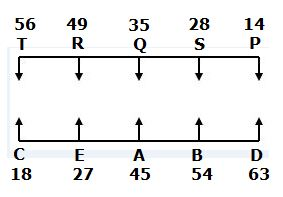• The one whose age is multiple of 5 faces the person who sits second to the right of C and either the one whose age is multiple of 5 or C sits at extreme ends of the line.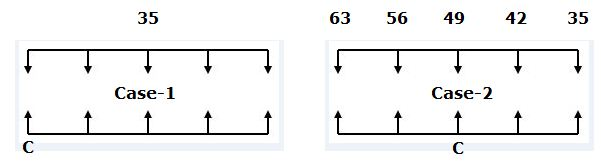• Only two persons are sitting between P and the one whose age is perfect square of a number. R faces the person who sits second to the left of B. Neither R nor B is the eldest persons in the each row.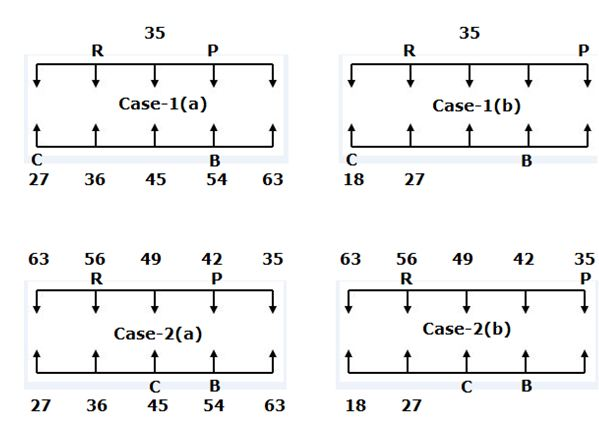• The difference between the ages of E and S is one year. E does not face S.
• So, Case-2(b) will be dropped.
• None of the two persons’ ages will be same.
• So, Case-2(a) will be dropped.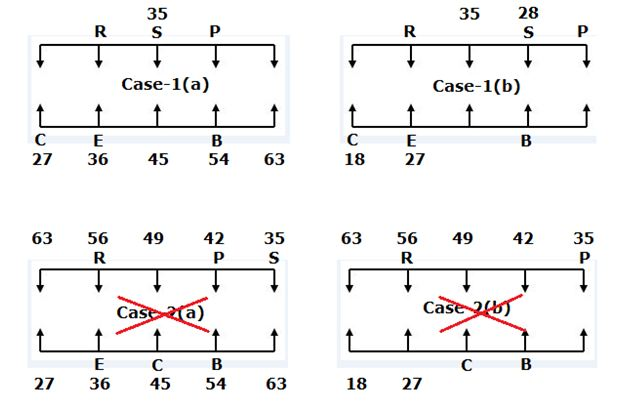• The difference between the ages of T and A is 11 years. R is 35 years elder than P.
• So, Case-1(a) will be dropped.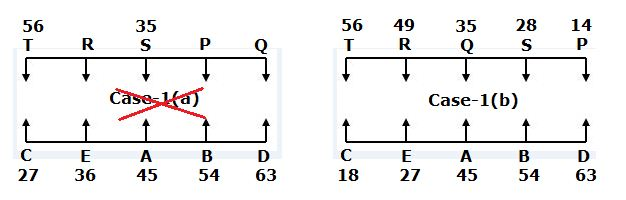Directions (6-10):

I. T%F –> T≥F –> T≥M=F –> True

II. K&V –> K<V –> K<T≥M≤J<V –> False

III. V\$B –> V>B –> V>J≥M≥B  –> True

I. J&N –> J<N –> J<S≤T=H≥N –> False

II. S\$R –> S>R –> S>J=A≥R –> True

III. A?H –> A≤H –> A=J<S≤T=H –> False

I. P\$V –> P>V –> P>L≥M>V –> True

II. R?T –> R≤T –> R≤V<M≤L≥T –> False

III. Q#T –> Q=L≥T  –> False

I. S&H –> S<H –> S≤D=F≤H –> False

II. A?R –> A≤R –> A<S≤D=F>R –> False

III. H#S –> H=S –> H≥F=D≥S –> False